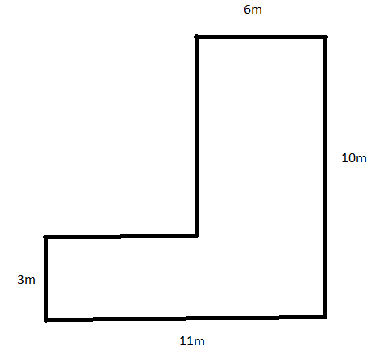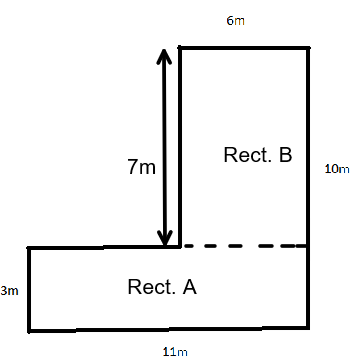Courses
Courses for Kids
Free study material
Offline Centres
MoreLast updated date: 25th Nov 2023
Total views: 278.7k
Views today: 4.78k

# The diagram shows a floor plan 10m not to scale. Calculate the area.Verified
278.7k+ views
Hint: According to the question, the area of irregular rectangles can be calculated in parts. As the above diagram is presented, we have to divide that figure in two regular shapes. We have to calculate the area of the two regular rectangles separately, and then add the final area that will be the final area of this irregular rectangle.
Formula used: $\text{area of rectangle} = \text{length} \times \text{breadth}$

Complete step-by-step solution:
Here we divide the given shape in two rectangles as:As the $\text{area of rectangle} = \text{length} \times \text{breadth}$
So, we will be finding the area of rectangle A
Given, $length = 3m$, $breadth = 11m$
So, $\text{area of rectangle} = \text{length} \times \text{breadth} = 3 \times 11 = 33$
Now, finding the area of rectangle B, we get:
Given, $length = 7m$, $breadth = 6m$
Calculating the area, we get:
$\text{area of rectangle} = \text{length} \times \text{breadth} = 7 \times 6 = 42$
Now, adding both the areas of rectangle A and rectangle B, we get:
$= 33 + 42 = 75$
So, the area of the irregular rectangle is 75 inches.

Note: A rectangle is a figure having four sides with four right angles. It has internal angles of ${90^ \circ }$. It is also called a four-sided polygon. The rectangle is always characterized in two parts. One is the length and the second one is the width. The size of the length and the width is always different. If their size is the same, then the figure is called a square instead of a rectangle.